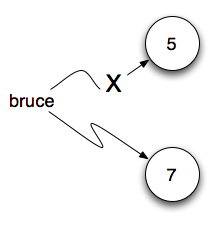# 2.10. Reassignment¶

As we have mentioned previously, it is legal to make more than one assignment to the same variable. A new assignment makes an existing variable refer to a new value (and stop referring to the old value).

The first time `bruce` is printed, its value is 5, and the second time, its value is 7. The assignment statement changes the value (the object) that `bruce` refers to.

Here is what reassignment looks like in a reference diagram:It is important to note that in mathematics, a statement of equality is always true. If `a is equal to b` now, then `a will always equal to b`. In Python, an assignment statement can make two variables refer to the same object and therefore have the same value. They appear to be equal. However, because of the possibility of reassignment, they don’t have to stay that way:

Line 4 changes the value of `a` but does not change the value of `b`, so they are no longer equal. We will have much more to say about equality in a later chapter.

## 2.10.1. Developing your mental model of How Python Evaluates¶

Its important to start to develop a good mental model of the steps Python takes when evaluating an assignment statement. In an assignment statement Python first evaluates the code on the right hand side of the assignment operator. It then gives a name to whatever that is. The (very short) visualization below shows what is happening.

a = 5
b = a

In the first statement a = 5 the literal number 5 evaluates to 5, and is given the name a. In the second statement, the variable a evaluates to 5 and so 5 now ends up with a second name b.

Note

In some programming languages, a different symbol is used for assignment, such as `<-` or `:=`. The intent is that this will help to avoid confusion. Python chose to use the tokens `=` for assignment, and `==` for equality. This is a popular choice also found in languages like C, C++, Java, and C#.